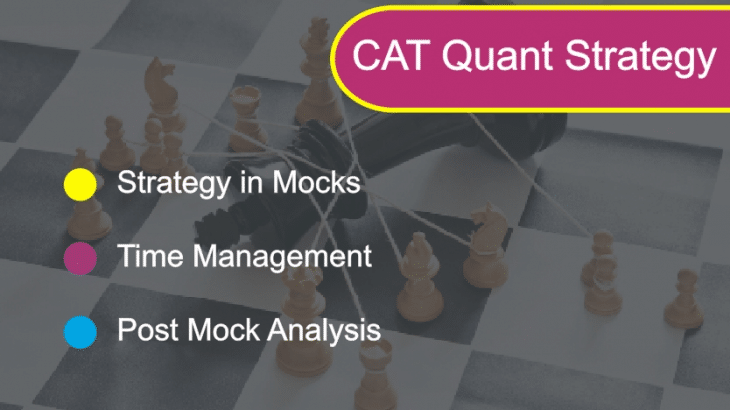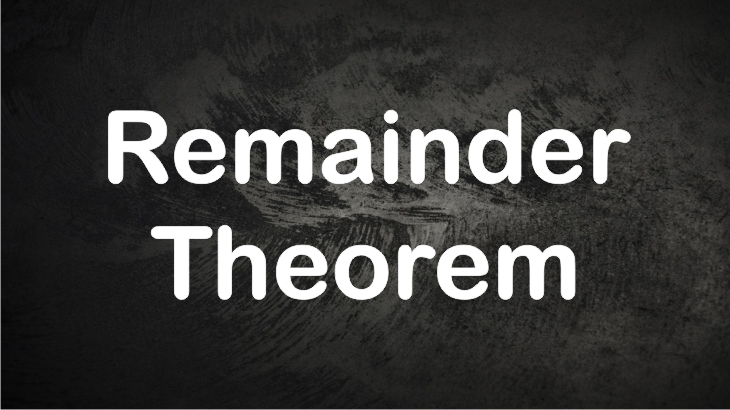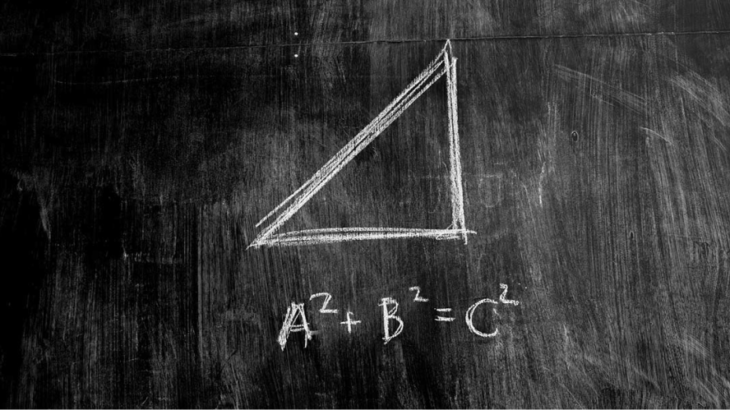## CAT Quant Mock Strategy and Analysis [CAT 2019]Before we discuss the mock strategy for CAT Quantitative Aptitude (QUANT) section, I would like to talk a little about the CAT 2018 Quantitative Aptitude pattern. This will help us prepare for this section, keeping in mind the most recent CAT exam. Topic No. of questions Toughness level Arithmetic 14 easy – moderate Algebra 10-11 […]

## CAT Geometry : An alternate approach to find areaA lot of questions in CAT and other management entrance exams are from Geometry and that too, from the topic called AREA. Many questions are based on finding the area of triangle, circles and quadrilaterals etc. Most of these questions can be solved in various ways. There is always a traditional method to solve such […]

## The right approach to learn Geometry for CAT Exam (Part -1)Geometry problems are an integral part of any aptitude based test like CAT. In recent CAT papers, around 7 questions have been consistently appearing in the quantitative aptitude section. I have been mentoring students for CAT exam over a decade. I have observed that each year, a good number of students face some peculiar problems […]

## Number of Factors Concepts and Formulas for CAT examNumber of factors based questions have regularly appeared in various competitive exams including CAT.  Primarily, these questions are based on the prime factorization of a number.  Generally,  factors based questions are of following types: The number of factors The sum of factors Product of factors Odd and Even Factors Number of Factors which are Perfect […]

## Logarithm Concepts Properties and Questions for CAT Exam

Logarithm and its properties are very vital concepts for solving many questions from algebra which appear in CAT and other MBA entrance exams. An aspirant who is preparing for CAT must be thorough with the basic properties of logarithm and its working while solving questions.  Let us understand the concepts of logarithm in detail. Definition of […]

## Remainder Theorem: Concepts | Tricks | Questions for CAT ExamRemainder theorem based number system questions are very famous among CAT aspirants. The reason for the same is that there are varieties of questions how to find remainders.  As a result, various theorems are used to solved problems on remainders. In this article, will deal with all the possible type of questions which frequently appear […]

## 7 Steps to Solve Logical Reasoning Puzzles for CAT Level ExamLogical reasoning puzzles which appear in the CAT exam are the most common types of questions in LRDI section. Unfortunately, we do not have any universally applicable method or approach to solve such puzzles. The reason is that these logical reasoning puzzles themselves have no standard structure. However,  an aspirant, after practicing lots of logical reasoning puzzle […]

## 3 Steps to solve Linear Arrangement Logical Reasoning for CAT ExamLinear arrangement based logical reasoning questions in CAT deal with sequentially aligning the objects by using the information given about their positions. In most of the cases, we have to identify the unique position of each of the objects or the relative position of one object compared to the other objects. Often, logical reasoning questions […]

## Relative Speed Concept and Questions for CAT Exam

Relative speed concept is can be of great help in solving many questions from time speed and distance in CAT exam. The concept is particularly critical for problems based on trains, and meeting points The idea is simple to understand, and we mainly need to know the working of the concept in the following cases Two bodies moving […]

## Pythagorean Triplets: Concepts Tricks and CAT ProblemsIf we scan the past CAT questions, then it is quite evident that most of the problems related to triangle required Pythagorean triplets as one of the concepts to solve them. It is such an essential theorem because it applies to a wide variety of questions. In this article will be dealing with the Pythagoras theorem […]